## Section8.7Conservation of Energy

In an earlier section, we found that coservative forces are special when it comes to work by them. We discovered that work by conservative forces is independent of the path between initial and final positions and can be written purely as a difference of potential energies at the beginning $U_i$ and at the end $U_f\text{.}$

\begin{equation*} W_{if}^{\text{conservative}} = U_i - U_f. \end{equation*}

Now, we ask: what will happen if only conservative forces are acting on a body? Replacing work in the work energy theorem will give us guidance to the answer.

\begin{equation} K_f - K_i = W_{if}^{\text{net}} = \sum W_{if}^{\text{conservative}} = \sum U_i - \sum U_f,\label{eq-work-energy-theorem}\tag{8.7.1} \end{equation}

where $\sum U_i$ is the sum of all potential energies, $U \text{,}$ evaluated at instant $t_i \text{,}$ and similarly for $\sum U_f \text{.}$ Rearranging terms in this equation we get

\begin{equation} K_i + \sum U_i = K_f + \sum U_f.\label{eq-kinetic-plus-potential-conserved}\tag{8.7.2} \end{equation}

That is, if all the forces are conservative, then the combination $K + \left( \sum U \right)$ is conserved during the motion.

We call the combination $K + \sum U$ energy (sometimes, mechanical energy), and denoted by symbol $E\text{.}$

\begin{equation} E = K + \sum U. \label{eq-definition-of-energy}\tag{8.7.3} \end{equation}

Equation (8.7.2) can now be expressed rather compactly as follows. If all forces acting on the body are conservative forces, then energy cannot change. This is called the principle of conservation of energy.

\begin{equation} E_i = E_f.\ \ \ \ (\text{all forces conservative})\label{eq-conservation-of-energy}\tag{8.7.4} \end{equation}

A skier starts near the top of a hill. His speed at a point on the slope that is $50\text{ m}$ above the sea level is $5.0\text{ m/s} \text{.}$

What will be his speed when he reaches a point that is $35\text{ m}$ above the sea level, assuming you can neglect the effect of drag and kinetic fricion forces on the skier?

Hint

Energy is conserved.

$17.9\text{ m/s}.$

Solution

Ignoring Drag and Kinetic Friction forces, the forces on the skier are (1) Weight and (2) Normal. Normal force will do no work since, at each instant, it is perpendicular to the displacement. Thus, we have only a conservative force on the skier. Therefore, his energy cannot change.

Equating the energy of the skier at the two instants in the problem statement,

\begin{equation*} \dfrac{1}{2}mv_f^2 + mgy_f = \dfrac{1}{2}mv_i^2 + mgy_i. \end{equation*}

The mass $m$ cancels out. Solving for $v_f$ we get

\begin{equation*} v_f = \sqrt{ v_i^2 + 2g(y_i - y_f) }. \end{equation*}

Now, we put in the numbers.

\begin{equation*} v_f = \sqrt{ 5.0^2 + 2\times 9.81\times ( 50 - 35) } = 17.9\text{ m/s}. \end{equation*}

A $200$-g steel ball is tied to a $2$-m “massless” string and hung from the ceiling to make a pendulum. The ball is released from rest at a point that makes a $30^{\circ}$ angle with the vertical direction. Find speed of the ball at the following points in its motion:

(a) vertically down,

(b) at an angle of $20^{\circ}$ with the vertical,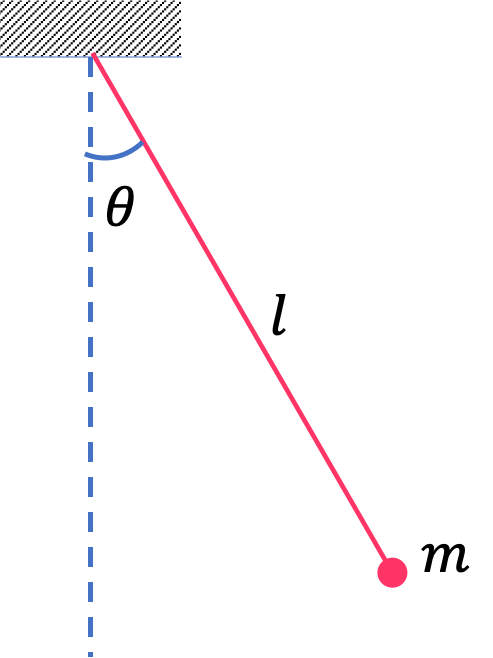Hint

Use energy conservation. For energy of pendulum bob, you need height from the bottom, $h = l - l \, \cos\, \theta \text{.}$

(a) $2.29 \text{ m/s}\text{,}$ (b) $1.7\text{ m/s}\text{.}$

Solution 1 (a)

The figure here shows the height $h$ of the bob from the zero potential energy reference. From the adjacent side of the triangle and the length of the string, we get

\begin{equation*} h = l - l \, \cos\, \theta. \end{equation*}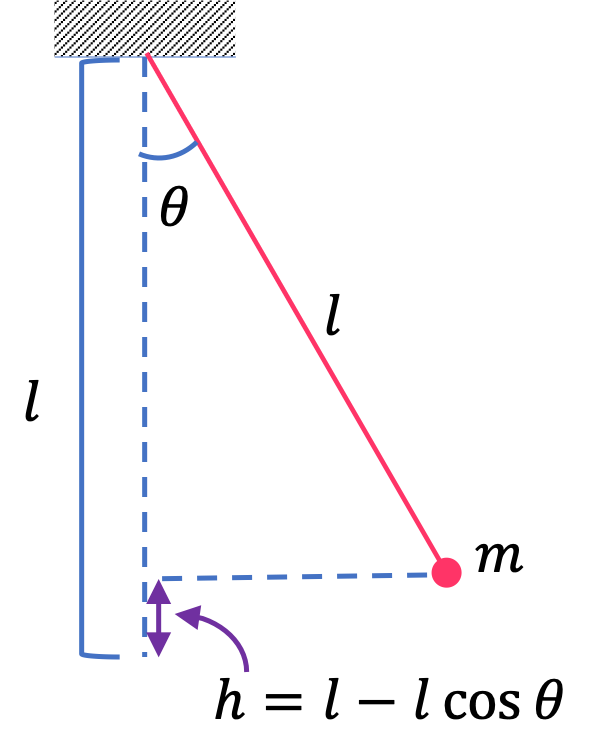At initial instant, the energy of the pendulum bob is all potential energy since it is at rest.

\begin{equation*} E = \dfrac{1}{2}mv^2 + mgh = 0 + 0.2\times 9.81\times 2\left(1-\cos\,30^\circ \right) = 0.5257\text{ J}. \end{equation*}

(a) Let $v_a$ stand for the speed when the bob is at the bottom of the swing. At this instant $h=0\text{.}$ Using the conservation of energy we get the following equation.

\begin{equation*} \dfrac{1}{2}mv_a^2 + 0 = 0.5257\text{ J}. \end{equation*}

Therefore,

\begin{equation*} v_a = \sqrt{ \dfrac{2\times 0.5257}{0.2} } = 2.29 \text{ m/s}. \end{equation*}
Solution 2 (b)

(b) Let $v_b$ stand for the speed when the bob is at the place when the pendulum angle is $20^\circ\text{.}$ Using the conservation of energy we get the following equation.

\begin{equation*} \dfrac{1}{2}mv_b^2 + mgh_b = E, \end{equation*}

where $E = 0.5257\text{ J}$ and

\begin{equation*} h_b = l \left(1 - \cos\, 20^\circ \right) = 0.1206\text{ m}. \end{equation*}

Solving for $v_b \text{.}$

\begin{equation*} v_b = \sqrt{ \dfrac{2}{m} \left( E - mg h_b\right)}. \end{equation*}

Numerically, we have

\begin{equation*} v_b = \sqrt{\dfrac{2}{0.2} (0.5257 - 0.2 \times 9.81 \times 0.1206 ) } = 1.7\text{ m/s}. \end{equation*}

A block of mass $300$ grams is attached to a spring of spring constant $100$ N/m. The other end of the spring is attached to a support while the block rests on a smooth horizontal table and can slide freely without any friction as illustrated in . The block is pushed horizontally till the spring compresses by $12$ cm, and then the block is released from rest.

(a) How much potential energy was stored in the block-spring-support system when the block was just released?

(b) Determine the speed of the block when it crosses the point when the spring is neither compressed nor stretched.

(c) Determine the speed of the block when it has traveled a distance of $20$ cm from where it was released.

Hint

(a) Use $U=\frac{1}{2}kx^2\text{.}$ (b) Use conservation of energy. (c) Use conservation of energy.

(a) $0.72\text{ J}\text{,}$ (b) $2.2\text{ m/s} \text{,}$ (c) $1.63 \text{ m/s}\text{.}$

Solution 1 (a)

(a) Using the formula for the spring potential energy we get

\begin{equation*} U_a = \dfrac{1}{2}kx^2 = \dfrac{1}{2}\times 100 \times 0.12^2 = 0.72\text{ J}. \end{equation*}
Solution 2 (b)

(b) When the block crosses the equilibrium point, $x = 0\text{.}$ That means potential energy is zero at this point. Let $v_b$ be the speed when passing through the equilibrium point (see Figure 8.7.5).

When the block was releases at the initial instant in part (a), all the energy was potential energy. Therefore, we get the following equation when we apply energy conservation.

\begin{equation*} \dfrac{1}{2} m v_b^2 + 0 = 0 + U_a. \end{equation*}

Therefore,

\begin{equation*} v_b = \sqrt{ \dfrac{2U_a}{m} } = \sqrt{ \dfrac{2 \times 0.72}{0.3} } = 2.2\text{ m/s}. \end{equation*}
Solution 3 (c)

(c) 20 cm from the place the motion began, the block would be 8 cm from the equilibrium, because it will go 12 cm to equilibrium and then stretch another 8 cm. Let's call this $x_c$ and the speed there as $v_c\text{.}$ Therefore, the energy conservation will give

\begin{equation*} \dfrac{1}{2} m v_c^2 + \dfrac{1}{2}kx_c^2 = 0 + U_a. \end{equation*}

Solving for $v_c$ gives

\begin{equation*} v_c = \sqrt{ \dfrac{2}{m} \left(U_a - \dfrac{1}{2}kx_c^2 \right) }. \end{equation*}

Now, we put the numbers.

\begin{equation*} \dfrac{1}{2}kx_c^2 = \dfrac{1}{2}\times 100 \times 0.08^2 = 0.32\text{ J}. \end{equation*}

Therefore,

\begin{equation*} v_c = \sqrt{\dfrac{2}{0.3}\left(0.72 - 0.32 \right) } = 1.63 \text{ m/s}. \end{equation*}

A block of mass $300$ grams is attached at the end of a massless spring of spring constant $10$ N/m. The other end of the spring is attached to the ceiling and the mass is brought to rest. Let us mark this point as O. Suppose, this point is taken to be the zero of the gravitational potential energy of the block. The zero of the spring potential energy is the point where the spring is neither stretched nor compressed, which is a distance $d$ above O.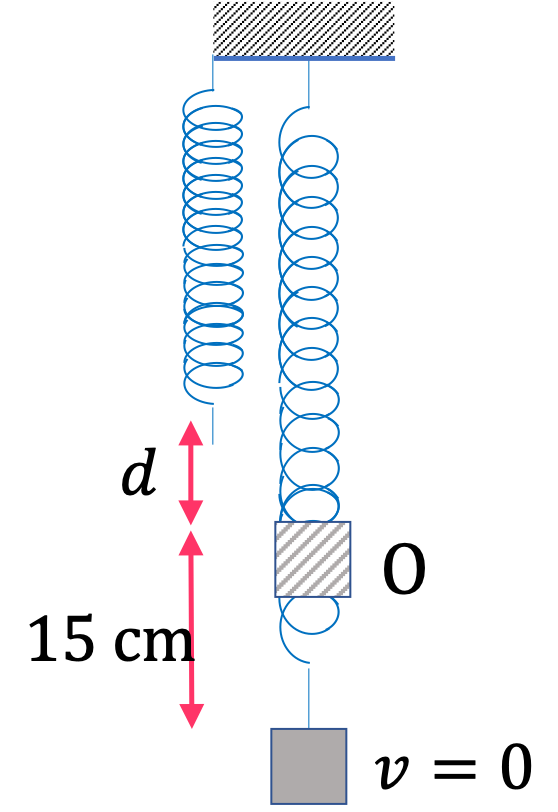The mass hangs freely and the spring is in a stretched state. The block is then pulled downward by another $15$ cm and released from rest. This will be the lowest point in the motion of the block since we are releasing the block from rest.

(a) How far above point O is the zero of the spring potential energy? That is, find $d\text{.}$

(b) What is the energy of the block at the instant the block is at the lowest point?

(c) What is the speed of the block at the instant the block returns to O?

Hint

(a) Balance forces at O. (b) Add two potential energies. (c) Use conservation of energy. (d) Use conervation of energy.

(a) $0.294 \text{ m} \text{,}$ (b) $0.55 \text{ J} \text{,}$ (c) $0.89 \text{ m/s}\text{.}$

Solution 1 (a)

(a) We just need to find the stretching that took place when we hung the block. Let this distance be $d\text{.}$ Balancing gravitational and spring forces when the block is at O gives

\begin{equation*} k d = mg. \end{equation*}

Therefore,

\begin{equation*} d = \dfrac{mg}{k} = \dfrac{0.3\times 9.81}{10} = 0.294 \text{ m}. \end{equation*}
Solution 2 (b)

(b) When the block is at the lowest point, it has only potential energy, which is a sum of the spring and gravitational potential energies. The net deformation of the spring is

\begin{equation*} \Delta l = 0.294 \text{ m} + 0.15 \text{ m} = 0.444\text{ m}. \end{equation*}

This will give

\begin{equation*} U_\text{sp} = \dfrac{1}{2}k (\Delta l)^2 = \dfrac{1}{2}\times 10 \times 0.444^2 = 0.99\text{ J}. \end{equation*}

The gravitational potential energy will be with respect to O. Since this point is below O, the gravitaional potential energy will be negative.

\begin{equation*} U_\text{gr} = mgh = 0.3\times 9.81\times (-0.15) = - 0.44\text{ J}. \end{equation*}

Therefore, the total energy at this point is

\begin{equation*} E_\text{bottom} = U_\text{sp} + U_\text{gr} = 0.55 \text{ J}. \end{equation*}
Solution 3 (c)

(c) Energy is conserved here. When the block returns to O, it has zero gravitational potential energy, but it still has spring potential energy for the stretch $d$ found in (a) above.

\begin{equation*} U_\text{sp,at O} = \dfrac{1}{2}\times 10 \times 0.294^2 = 0.432 \text{ J}. \end{equation*}

Applyig energy conservation between this point and the lowest point of motion in part (b) gives the following for speed $v$ when at O.

\begin{equation*} \dfrac{1}{2}mv^2 + U_\text{sp,at O} + 0 = E_\text{bottom}. \end{equation*}

This gives

\begin{equation*} v = \sqrt{ \dfrac{ 2(0.55 - 0.432) }{0.3} } = 0.89 \text{ m/s}. \end{equation*}

A block of mass $500\text{ grams}$ is attached to a spring. The other end of the spring is attached to a support while the mass rests on a smooth frictionless surface inclined at angle of $30^{\circ}\text{.}$ Initially, when the block is resting, the spring is compressed by $2 \text{ cm}\text{.}$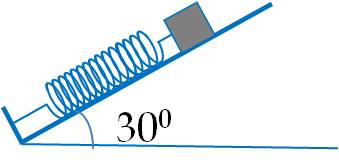The block is pushed along the surface till the spring compresses by additional $10 \text{ cm}$ and then released from rest. After that, the block executes a back and forth motion along the inclined surface.

(a) What is the spring constant of the spring?

(b) (i) How much potential energy was stored in the block-spring-support system at the instant the block was released? (ii) Taking this point to be the zero of the gravitational potential energy, what is the total energy of the block when it is at this location?

(c) Determine the speed of the block when it crosses the point when the spring is neither compressed nor stretched.

(d) Determine the position of the block where it just comes to rest on its way up the incline. Express the distance of this point on the incline from the point it was released.

Hint

(a) $123\text{ N/m}\text{,}$ (b) $0.883\text{ J}\text{,}$ (c) $1.54\text{ m/s}\text{,}$ (d) $0.20\text{ m}\text{.}$

Solution 1 (a)

We balance forces along the incline when the block is not yet pushed. The component of weight along the incline is balanced by the spring force from compression by $2\text{ cm}\text{.}$

\begin{align*} kx \amp = mg\sin\theta \\ \amp = 0.5\times 9.81\times \sin\,30^\circ = 2.45\text{ N}. \end{align*}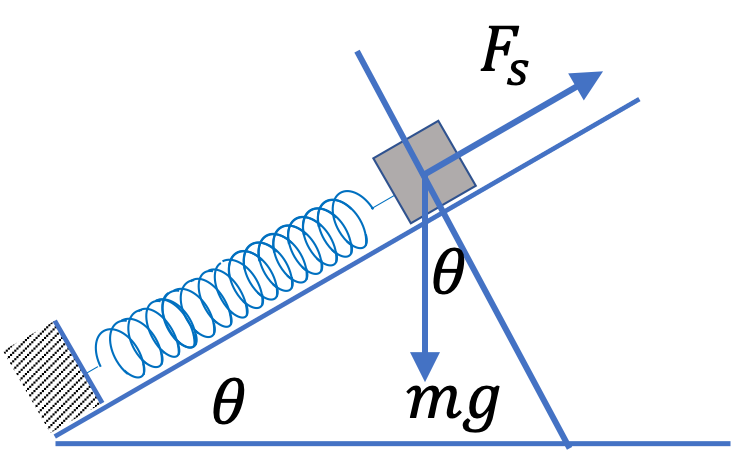Therefore,

\begin{equation*} k = \dfrac{2.45\text{ N}}{0.02\text{ m}} = 123\text{ N/m}. \end{equation*}
Solution 2 (b)

(b) (i) Spring potential energy with $\Delta l = 0.12\text{ m}$ gives the answer. Let's refer to this point as b.

\begin{equation*} U_\text{sp,b} = \dfrac{1}{2}kx^2 = \dfrac{1}{2}\times 122.6 \times 0.12^2 = 0.883\text{ J}. \end{equation*}

(ii) Based on the choice of zero of gravitational potential energy, we have

\begin{equation*} U_\text{gr,b} = 0 \end{equation*}

Therefore, potential energy of the block when it is at this location is

\begin{equation*} U_\text{b} = 0.883+0 = 0.883\text{ J}. \end{equation*}

Since the block is at rest here, $K_\text{b} = 0\text{.}$ Therefore, total energy of the block is

\begin{equation*} E_\text{b} = 0.883\text{ J}. \end{equation*}
Solution 3 (c)

At this point $U_\text{sp,c} = 0\text{,}$ but we have gravitaional potential energy nonzero.

\begin{equation*} U_\text{gr,b} = mgh_c, \end{equation*}

where $h_c$ is the vertical height of this point from the point where we choose the zero of the gravitational potential energy. The two points are separated by $0.12\text{ m}$ along the incline. That means

\begin{equation*} h_c = 0.12\,\sin\,30^\circ = 0.06\text{ m}. \end{equation*}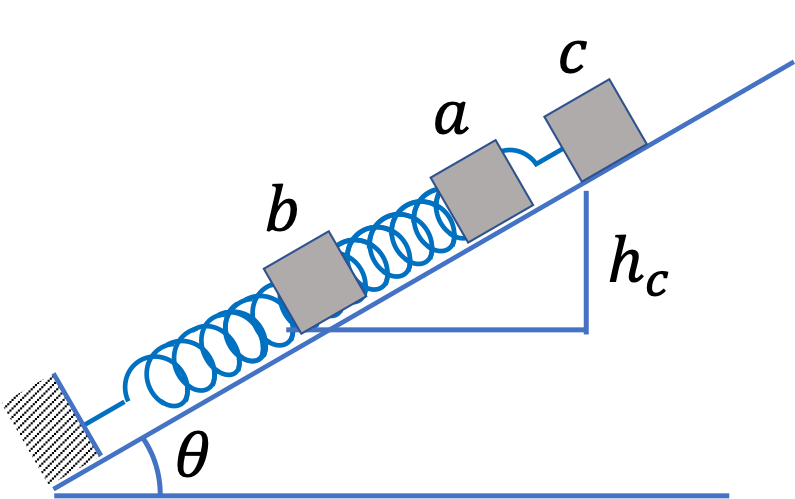Therefore,

\begin{equation*} U_\text{gr,c} = mgh_c = 0.5\times 9.81\times 0.06 = 0.294\text{ J}. \end{equation*}

Using conservation of energy between (b) and this point we get

\begin{equation*} \dfrac{1}{2}mv_c^2 + 0.294 = 0.883. \end{equation*}

This gives

\begin{equation*} v_c = \sqrt{ 2( 0.883 - 0.294 )/0.5} = 1.54\text{ m/s}. \end{equation*}
Solution 4 (d)

Let $x$ denote the distance on the incline the block moved from the point it was released. This would mean the spring would be extended by

\begin{equation*} \Delta l = x - 0.12, \end{equation*}

and height $h_d$ of this point is

\begin{equation*} h_d = x\,\sin\,30^{\circ} = \dfrac{x}{2}. \end{equation*}

Now, setting up conservation of energy with total energy $0.883\text{ J}$ gives

\begin{equation*} \dfrac{1}{2}k\left( x - 0.12\right)^2 + m g \dfrac{x}{2} = 0.883. \end{equation*}

Solving this, keeping the positive root gives

\begin{equation*} x = 0.20\text{ m}. \end{equation*}

A satellite of mass $m$ is to be placed in a circular orbit of radius $2 R_E\text{,}$ where $R_E$ is the radius of the Earth. With what speed with respect to the fixed center of the Earth should the satellite be launched from a point on the equator of the Earth?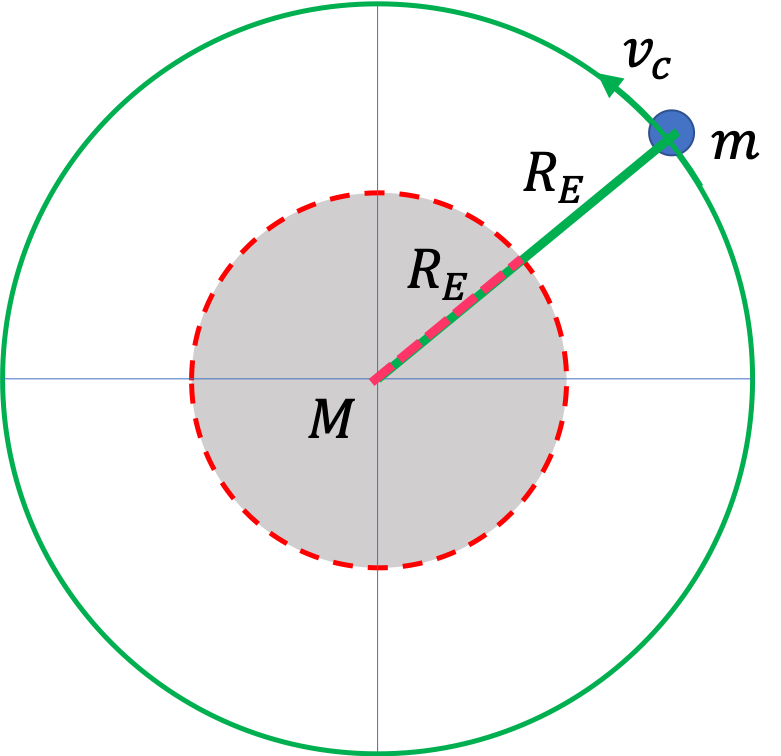Hint

First find the speed when in the circular orbit by using Newton's equation for uniform circular motion. Then, find the energy when in the circular orbit. Finally, use energy conservation to find the speed of launch.

$\sqrt{ \dfrac{3 G M_E}{4 R_E } } \approx 6.8\text{ km/s}\text{.}$

Solution

Strategy: Let $v_0$ be the speed of launch and $v_c$ the speed when the satellite is in the circular orbit. We will first obtain $v_c$ from Newton's equation of motion for the circular motion. Then, we will get energy of the satellite when in the orbit. After that, energy conservation between energy when in circular orbit and immediately after lauch will give us a condition from which we will obtain $v_0\text{.}$

The equation of motion of the circular orbit for uniform circular motion gives

\begin{equation*} m\,\dfrac{v_c^2}{ 2R_E } = G \dfrac{M_E m}{ \left(2 R_E \right)^2 }. \end{equation*}

Solve this for $\dfrac{1}{2}mv_c^2$ to get

\begin{equation*} \dfrac{1}{2} m v_c^2 = G \dfrac{M_E m}{ 4 R_E }. \end{equation*}

To this we add the potential energy to get the energy $E_c$ when the satellite is in the circular orbit.

\begin{equation*} E_c = \dfrac{1}{2} m v_c^2 - G \dfrac{M_E m}{ 2 R_E } = - G \dfrac{M_E m}{ 4 R_E }. \end{equation*}

The energy $E_0$ at the instant after launch is

\begin{equation*} E_0 = \dfrac{1}{2} m v_0^2 - G \dfrac{M_E m}{ R_E }. \end{equation*}

Equating $E_0$ and $E_c$ we get

\begin{equation*} \dfrac{1}{2} m v_0^2 - G \dfrac{M_E m}{ R_E } = - G \dfrac{M_E m}{ 4 R_E }. \end{equation*}

Therefore,

\begin{equation*} v_0 = \sqrt{ \dfrac{3 G M_E}{2 R_E } } \approx 6.8\text{ km/s}. \end{equation*}

A spacecraft of mass $m$ is launched vertically upward from the surface of the Earth (radius $R_E$) with initial speed $v_0\text{.}$ Assume only gravitation of Earth acts on the spacecraft. (a) Find an expression of maximum distance $r_\text{max}\text{,}$ the spacecraft will go before coming down, assuming $v_0$ is not sufficient to escape the gravity of Earth? (b) What is the minimum value of $v_0$ at which spacecraft will escape the gravity of Earth? (c) If spacecraft has mass $100\text{ kg}\text{,}$ how much energy would be needed to escape the gravity of Earth?

Hint

(a) Use Conservation of energy using $U=-GMm/r\text{.}$

(a) $\frac{2gR_E^2}{ 2gR_E - v_0^2 }\text{,}$ (b) $\sqrt{2gR_E}\text{,}$ $1.12 \times 10^{4}\text{ m/s}\text{,}$ (c) $6.25\times 10^{9}\text{ J}\text{.}$

Solution 1 (a)

(a) Since only gravitational force is acting, energy of the spacecraft after launch will be conserved. At the maximum distance point, speed of the craft will be zero.

Thus, we get

\begin{equation} \frac{1}{2}mv_0^2 - G_N \frac{M_E m}{R_E} = 0 - G_N \frac{M_E m}{r_\text{max}}. \label{eq-spacecraft-escape-velocity-energy-conservation}\tag{8.7.5} \end{equation}

Also, note that $g$ of Earth also relates to its mass and radius by

\begin{equation*} g = G_N \frac{M_E }{R_E^2}. \end{equation*}

Using this in Eq. (8.7.5) we get

\begin{equation*} \frac{1}{2}mv_0^2 - mgR_E = - m g R_E^2 \frac{1}{r_\text{max}}. \end{equation*}

This can be solved for $r_\text{max}\text{.}$

\begin{equation} r_\text{max} = \frac{2gR_E^2}{ 2gR_E - v_0^2 }.\label{eq-spacecraft-escape-velocity-max-distance}\tag{8.7.6} \end{equation}
Solution 2 (b)

(b) To escape means $r_\text{max} = \infty\text{.}$ From Eq. (8.7.6), we see that it will occur when

\begin{equation*} 2gR_E - v_0^2 = 0. \end{equation*}

Lets denote this initial speed by $v_\text{es}\text{.}$

\begin{equation} v_\text{es} = \sqrt{2gR_E}.\label{eq-spacecraft-escape-velocity-formula}\tag{8.7.7} \end{equation}

With $g=9.81\text{ m/s}^2\text{,}$ $R_E=6.37\times10^6\text{ m}\text{,}$ we get

\begin{equation*} v_\text{es} = 1.12 \times 10^{4}\text{ m/s}. \end{equation*}
Solution 3 (c)

(c) The energy needed will be the kinetic energy at the launch site.

\begin{equation*} E = \frac{1}{2}m v_\text{es}^2 = m g R_E. \end{equation*}

Now, we use numerical values to get

\begin{equation*} E = 6.25\times 10^{9}\text{ J}. \end{equation*}